Worksheet: How Many Squares?

# Worksheet: How Many Squares? Notes | Study Mathematics for Class 5: NCERT - Class 5

## Document Description: Worksheet: How Many Squares? for Class 5 2022 is part of Mathematics for Class 5: NCERT preparation. The notes and questions for Worksheet: How Many Squares? have been prepared according to the Class 5 exam syllabus. Information about Worksheet: How Many Squares? covers topics like and Worksheet: How Many Squares? Example, for Class 5 2022 Exam. Find important definitions, questions, notes, meanings, examples, exercises and tests below for Worksheet: How Many Squares?.

Introduction of Worksheet: How Many Squares? in English is available as part of our Mathematics for Class 5: NCERT for Class 5 & Worksheet: How Many Squares? in Hindi for Mathematics for Class 5: NCERT course. Download more important topics related with notes, lectures and mock test series for Class 5 Exam by signing up for free. Class 5: Worksheet: How Many Squares? Notes | Study Mathematics for Class 5: NCERT - Class 5
 1 Crore+ students have signed up on EduRev. Have you?

Q.1. Draw 2 straight lines in this rectangle to divide it into 1 rectangle and 2 equal triangles. Find the area of the new rectangle and the area of 1 of the triangles.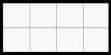Q.2. Write the area in square cm of the shaded portion.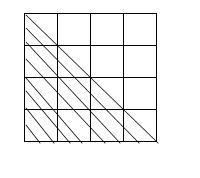Q.3. Find the perimeter and area of the following figure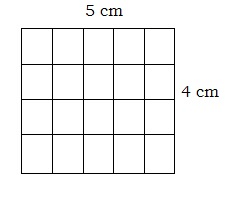Q.4.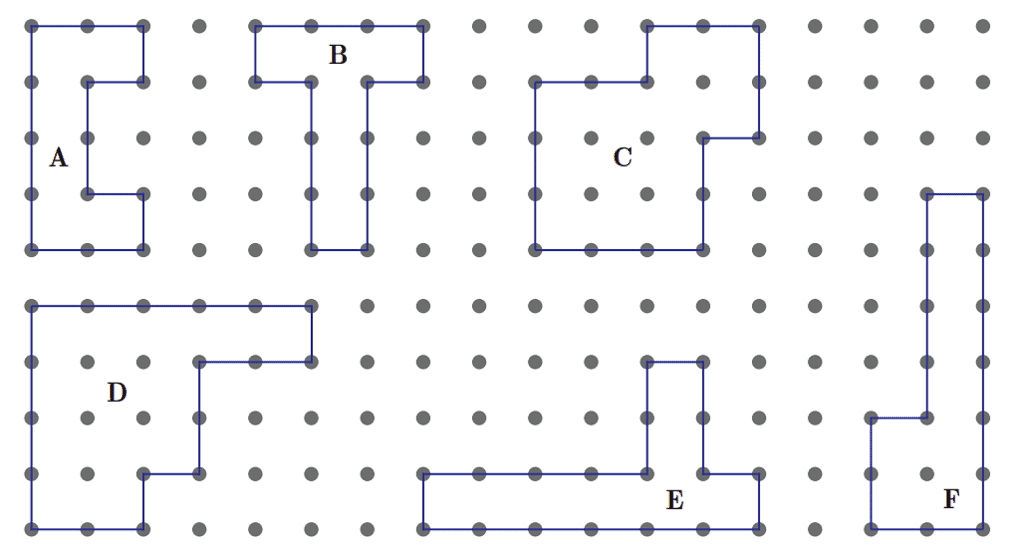(a)  Which shape has the maximum perimeter? Its perimeter is?

(b) Which shape has the minimum perimeter? Its perimeter is

(c) The perimeter of figure D is____cm. It is _____ (less/more) than the perimeter of figure A which is______ cm

Q.5. Study the diagram given below. Draw 2 more lines so that the area is 18 square cm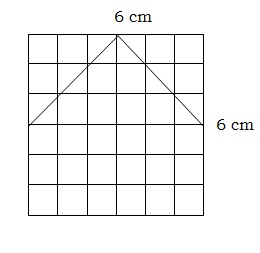Q.6. Here is a rectangle of an area of 20 square cm.

a). Draw one straight line in this rectangle to divide it into two equal triangles. What is the area of each of the triangles?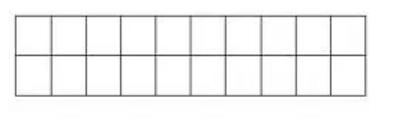b) Draw one straight line in this rectangle to divide it into two equal rectangles. What is the area of each of the smaller rectangles?

c) Draw two straight lines in this rectangle to divide it into one rectangle and two equal triangles. What is the area of the rectangle? What is the area of each of the triangles?

Q.7. Find the perimeter and area of each rectangle.
(a)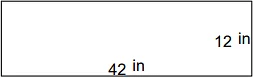(b)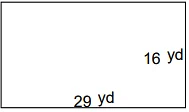(c)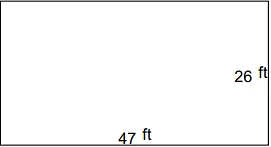(d)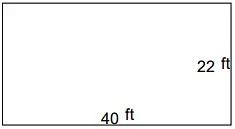(e)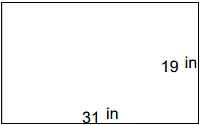(f)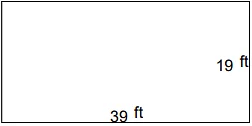(g)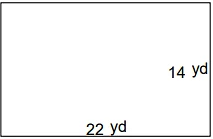(h)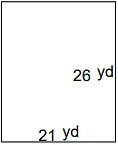(i)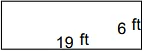(j)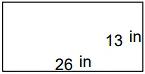Q.8.
(a) The area of a square is 9 cm. Find the area of shaded part: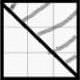(b) Find the perimeter of the following figure: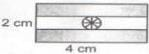(c) Find the area of the shaded shape: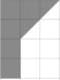(d) Find the area of the following: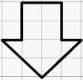(e) Find the area of the shaded path.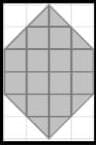The document Worksheet: How Many Squares? Notes | Study Mathematics for Class 5: NCERT - Class 5 is a part of the Class 5 Course Mathematics for Class 5: NCERT.
All you need of Class 5 at this link: Class 5

## Mathematics for Class 5: NCERT

33 videos|59 docs|39 tests
 Use Code STAYHOME200 and get INR 200 additional OFF

## Mathematics for Class 5: NCERT

33 videos|59 docs|39 tests

Track your progress, build streaks, highlight & save important lessons and more!

,

,

,

,

,

,

,

,

,

,

,

,

,

,

,

,

,

,

,

,

,

;